### 作业描述### 读取数据

``````def readfile(path, label):
# label 是一個 boolean variable，代表需不需要回傳 y 值
image_dir = sorted(os.listdir(path))
x = np.zeros((len(image_dir), 128, 128, 3), dtype=np.uint8)
y = np.zeros((len(image_dir)), dtype=np.uint8)
for i, file in enumerate(image_dir):
x[i, :, :] = cv2.resize(img,(128, 128))
if label:
y[i] = int(file.split("_"))
if label:
return x, y
else:
return x
workspace_dir = './data'
train_x, train_y = readfile(os.path.join(workspace_dir, "training"), True)
print("Size of training data = {}".format(len(train_x)))
val_x, val_y = readfile(os.path.join(workspace_dir, "validation"), True)
print("Size of validation data = {}".format(len(val_x)))
print("Size of Testing data = {}".format(len(test_x)))``````

### 设计 CNN

``````model = keras.models.Sequential()
# the raw shape (128,128,3)
# This time the shape is (32,128,128,3)
# MaxPooling
# （32,64,64,3）
#  (64,32,32,3)
# Flatten and input Neural NetWOrk; set activation = selu(auto_batch_normalization)
# 20 layers; 100 nodes
for _ in range(20):

metrics = ['accuracy'])``````

image data先经过一次Convolution，我设计了 32 个filter，每个是（3，3）的矩阵，至于为什么选这个数，大家都喜欢用（具体原因我还在研究这篇文章），再经过一层MaxPooling，这里也用常用的（2，2），再来一组这样的操作，只不过这时候的filter的个数变成了原来的 2 倍。

### 训练网络

#### 第一次训练

``````logdir = './dnn_callbacks'
if not os.path.exists(logdir):
os.mkdir(logdir)
output_model_file = os.path.join(logdir,
"cnn_classfication_model.h5")
callbacks = [
keras.callbacks.TensorBoard(logdir),
keras.callbacks.ModelCheckpoint(output_model_file,
save_best_only=True),
]
history = model.fit(train_x, train_y, epochs=10,
callbacks=callbacks)``````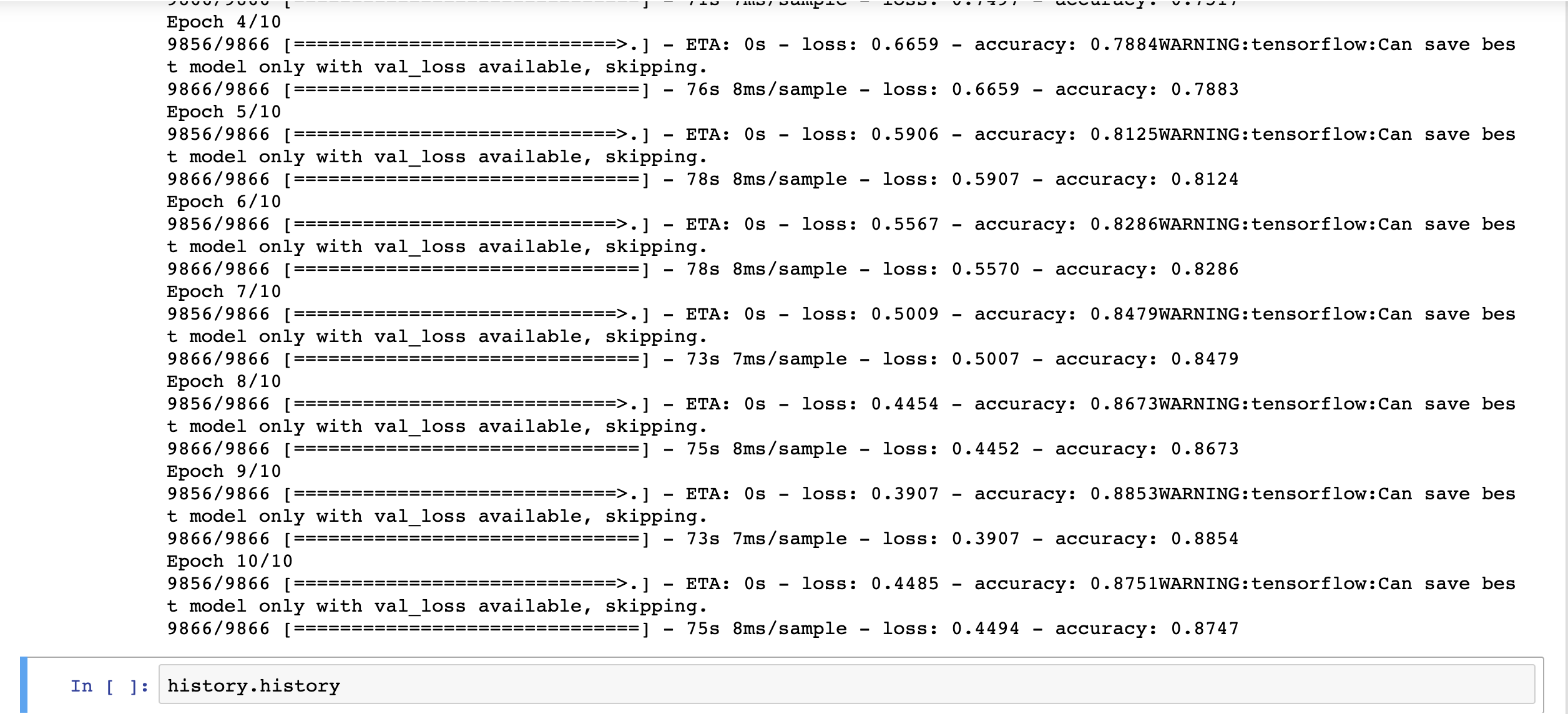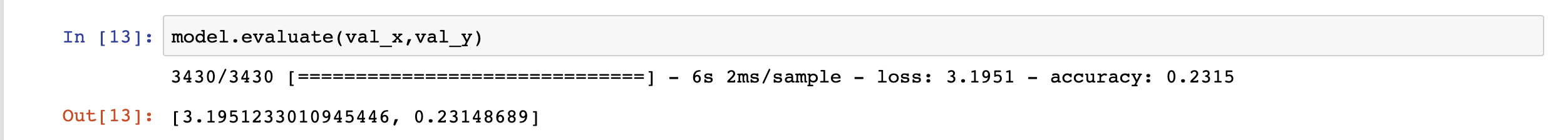• Early Stoping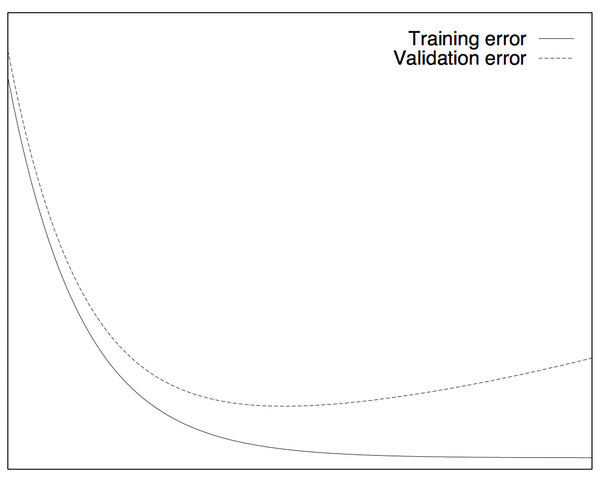• Dropout

• Regularization（正则化）

#### 第二次训练 - 加上 Dropout

``````model = keras.models.Sequential()
for _ in range(20):
metrics = ['accuracy'])``````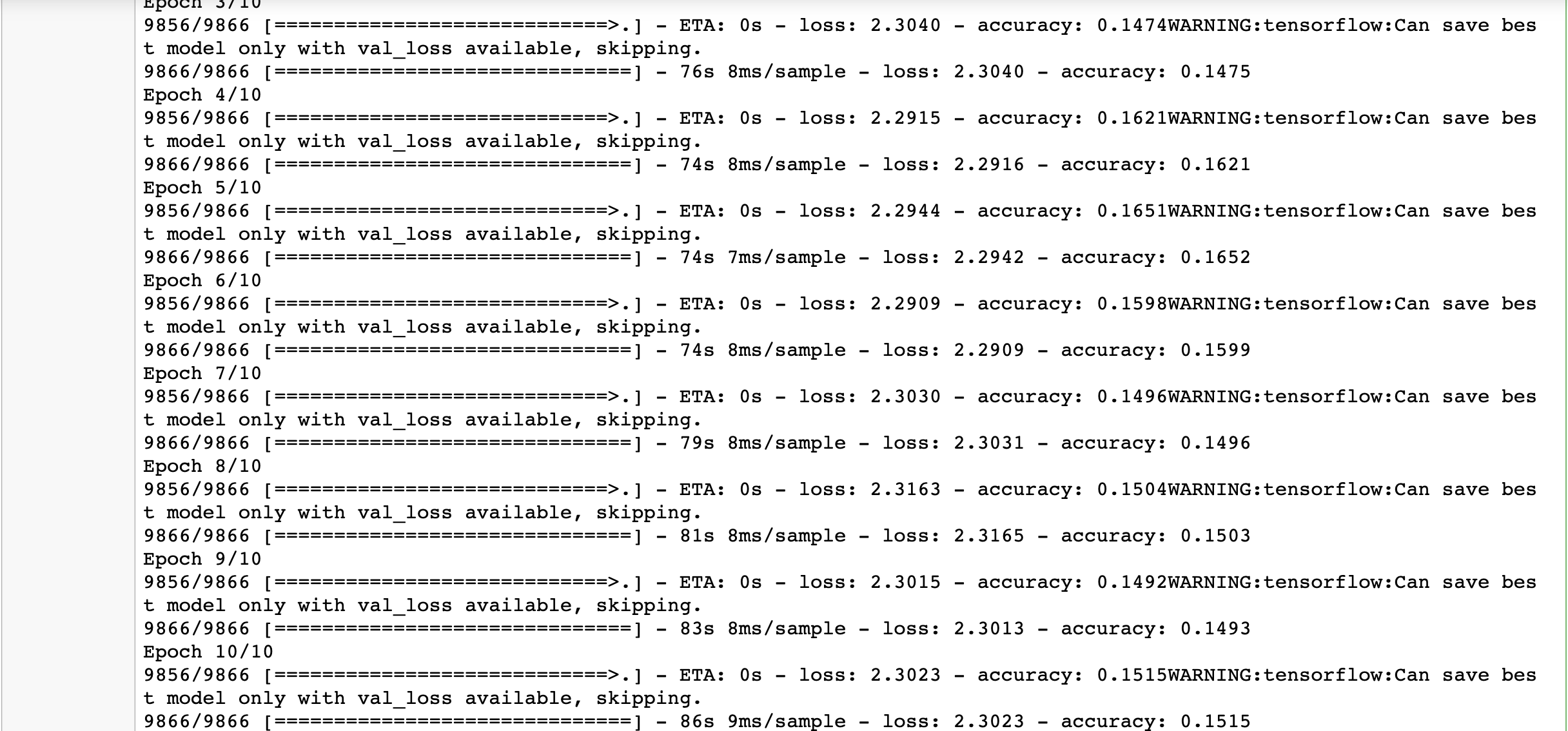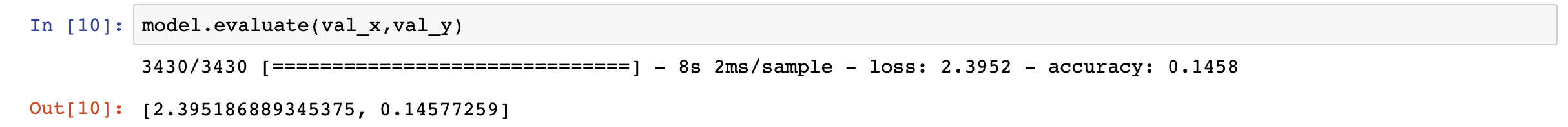#### 第三次训练 - 加上 Early Stopping

``````logdir = './dnn_callbacks'
if not os.path.exists(logdir):
os.mkdir(logdir)
output_model_file = os.path.join(logdir,
"cnn_classfication_model.h5")
callbacks = [
keras.callbacks.TensorBoard(logdir),
keras.callbacks.ModelCheckpoint(output_model_file,
save_best_only=True),
keras.callbacks.EarlyStopping(patience=5,min_delta=1e-3) # 这里是新增的内容
]
history = model.fit(train_x, train_y, epochs=10,
callbacks=callbacks)``````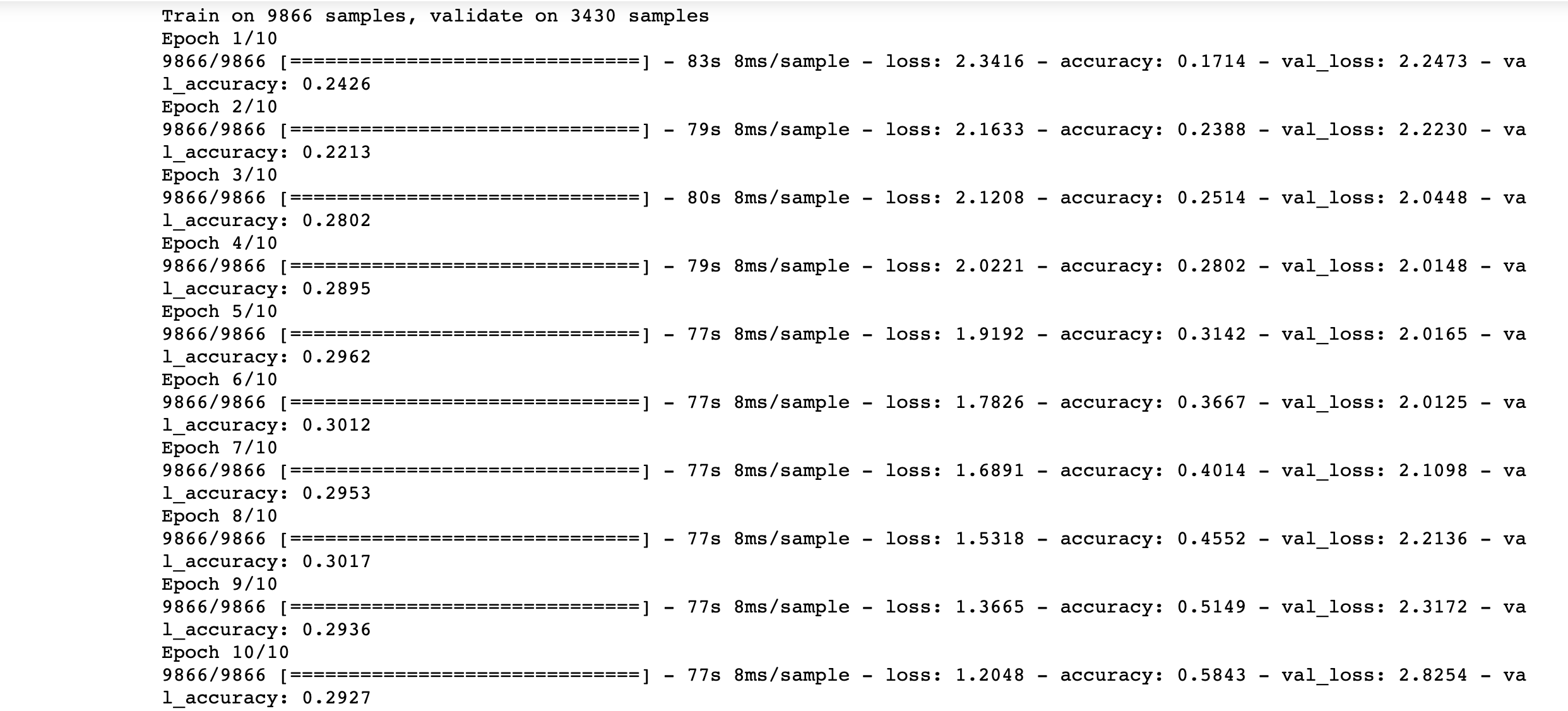Early Stopping 并没有发挥作用，也就是说，即使我们不加 Early Stopping，仅加上验证集，也就会得到这样的结果，真是蛋疼。

• New Activation Function

我认为，这个不需要考虑了吧，还有什么比 selu 更适合的呢。